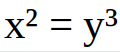# Mathematical sbi contest #5Here you can keep your brain fit by solving math related problems and also earn SBI.

To everyone who already participated in a past contest, come back today and try a new problem(tell me if you don't want to be tagged):
@addax @ajayyy @bwar @heraclio @sidekickmatt @tonimontana @vote-transfer

In case no one gets a result(which I doubt), I will give away the 1 SBI to anyone who comments.

This contest is over! You can find the results here.

I don’t know the answer but here’s giphy’s auto response... !giphy-auto

Posted using Partiko iOS

23.08.2019 08:38
1// You can support giphy by using one of your witness votes on untersatz! //

23.08.2019 08:38
0

Why do many people react to a math equation with something like
`"OMG! What kind of alien language is that?"`, instead of just saying
`"Wow, that's easy. The solution is …"`?

Even if you got no idea how to work with an equation, you should be able to just plug some random numbers in for x and y until you get to the result.

23.08.2019 09:05
3// You can support giphy by using one of your witness votes on untersatz! //

23.08.2019 09:05
0

Haha! OK.

How about if `x = 1` and `y = 1`?

`1^2 = 1^3` (1 = 1).

23.08.2019 09:13
1

Oh, I will take the easy answer
x=1, y=1
Doing math in my head is easy some days, hard other days. Power of three not so easy.

23.08.2019 15:41
1

x=8,y=4
x=27,y=9
x=64,y=16
x=125, y=25
x=216, y=36
x=z^3,y=z^2 pattern continues for ever so I'd like to submit every answer up to and including infinity.

23.08.2019 21:00
1

To get solutions of x^2=y^3 one can look for solutions of y=x^(2/3). Taking x=t^3 for some other integer t one can guarantee that y=t^(3*2/3)=t^2 is an integer. So my solutions would be
x=0,y=0
x=1,y=1
x=-1,y=1
x=8,y=4
x=-8,y=4
...

24.08.2019 18:09
0Here is a free 100% upvote from @contestkings that has a balance of 32,000 SP + 10,500 PAL + 310,000 SPT + 45,000 BATTLE +32,000 NEOXAG.

If you run a contest and need our free community support click here to connect with us today!

24.08.2019 22:57
6

This post was resteemed by @contrabourdon!

Send a min of 1 STEEM/SBD with post in memo for a resteem!

// You can support minnowresteemr by using one of your witness votes on untersatz! //

25.08.2019 00:10
0